## two loudspeakers facing each other are separated by 1.00 m and are excited in phase by a 1,100 Hz sine wave. Where will be the points on a l

Question

two loudspeakers facing each other are separated by 1.00 m and are excited in phase by a 1,100 Hz sine wave. Where will be the points on a line between speakers where there will be constructive interference, and where will be the points where there will be destructive interference

in progress 0
3 weeks 2021-08-23T23:44:17+00:00 1 Answers 0 views 0

Condition of constructive interference is given as

x = 95 cm, 80 cm, 65 cm, 50 cm, 35 cm, 20 cm, 5 cm

condition of destructive interference we know that

x = 87.5 cm, 72.5 cm, 57.5 cm, 42.5 cm, 27.5 cm, 12.5 cm

Explanation:

As we know that the frequency of the sound waves is 1100 Hz

now we can find the wavelength as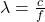now we have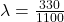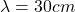now we know for the condition of constructive interference

Path difference = N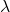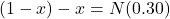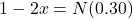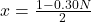x = 95 cm, 80 cm, 65 cm, 50 cm, 35 cm, 20 cm, 5 cm

Similarly for condition of destructive interference we know that

Path difference = (2N + 1)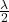so we have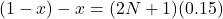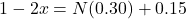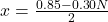x = 87.5 cm, 72.5 cm, 57.5 cm, 42.5 cm, 27.5 cm, 12.5 cm